• fir数字滤波器matlab代码
千次阅读
2021-08-06 09:21:28

### 目录：

本文是基于MATLAB的数字滤波器设计，所有数据基于计算机内部处理，因而都是离散信号，所以采用的是数字滤波器。从实现的网络结构或者单位脉冲响应来看，数字滤波器可以分为FIR(Finite Impulse Response , 有限脉冲响应)滤波器和IIR(Infinite Impulse Response , 无线脉冲响应)滤波器。本文主要从两个方面介绍FIR滤波器的MATLAB设计：集成函数和工具箱。

## 1 FIR滤波器的原理

从时域看，FIR的一般表达式H(z)如下：

∑ n = 0 N − 1 h ( n ) z − n = h ( 0 ) + h ( 1 ) z − 1 + . . . + h ( N − 1 ) z − ( N − 1 ) \sum_{n=0}^{N-1}h(n)z^{-n}=h(0)+h(1)z^{-1}+...+h(N-1)z^{-(N-1)}

从表达式至少可以看出两点：系统只在原点处有极点，可以用抽头法构建模型，每一个乘法器的系数即为抽头系数。
由于N是有限值，因此抽头系数项是有限的，可以得到h(n)：

∑ n = 0 N − 1 h ( 0 ) δ ( n ) + h ( 1 ) δ ( n − 1 ) + . . . + h ( N − 1 ) δ ( n − ( N − 1 ) ) \sum_{n=0}^{N-1}h(0)δ(n)+h(1)δ(n-1)+...+h(N-1)δ(n-(N-1))

可以看出h(n)，即系统的脉冲响应的项数是有限的，因此成为有限脉冲响应。

## 2 FIR滤波器的特点

FIR一个突出的优点就是具有严格的线性相位特性，但并不是所有结构的FIR都具备此特性，只有当FIR滤波器的单位脉冲响应满足对称条件时，FIR才具有线性相位特性。

### 2.1 相位特性

研究FIR的相位特性时，将结构分为奇对称和偶对称两种。

#### 2.1.1 偶对称

当FIR单位脉冲响应满足偶对称，即有：

h ( n ) = h ( M − n ) , 0 ≤ n ≤ M h(n)=h(M-n), 0 ≤ n ≤ M

代入H(z)的表达式：

∑ n = 0 M h ( n ) z − n = ∑ n = 0 M h ( M − n ) z − n \sum_{n=0}^{M}h(n)z^{-n}=\sum_{n=0}^{M}h(M-n)z^{-n}

令M-n=k , 对等号右边部分做变换：

H ( z ) = ∑ k = 0 M h ( k ) z − ( M − k ) = z − M ∑ n = 0 M h ( k ) z k = z − M H ( z − 1 ) H(z)=\sum_{k=0}^{M}h(k)z^{-(M-k)}=z^{-M}\sum_{n=0}^{M}h(k)z^k=z^{-M}H(z^{-1})

所以H(z)有两种表达方式，那么H(z)可以表示为：

H ( z ) = 1 2 [ H ( z ) + z − M H ( z ) ] = 1 2 ∑ k = 0 M h ( n ) [ z − n + z − M z n ] H(z)=\frac{1}{2}[H(z)+z^{-M}H(z)]=\frac{1}{2}\sum_{k=0}^{M}h(n)[z^{-n}+z^{-M}z^{n}]

提取一项 z − M 2 z^{-\frac{M}{2}} ，可得到滤波器频响：

H ( e j w ) = e − j w M 2 ∑ n = 0 M h ( n ) c o s [ w ( M 2 − n ) ] = A ( w ) e − j w M 2 H(e^{jw})=e^{-jw\frac{M}{2}}\sum_{n=0}^{M}h(n)cos[w(\frac{M}{2}-n)]=A(w)e^{-jw\frac{M}{2}}

根据相频定义，可知FIR相频为：

φ ( w ) = − M 2 w φ(w)=-\frac{M}{2}w

故满足线性相位特性。

#### 2.1.2 奇对称

当FIR单位脉冲响应满足偶对称，即有：

h ( n ) = − h ( n − M ) , 0 ≤ n ≤ M h(n)=-h(n-M), 0 ≤ n ≤ M

由2.1.1推导思路可得：

H ( e j w ) = e − j w M 2 + π 2 ∑ n = 0 M h ( n ) s i n [ w ( M 2 − n ) ] = A ( w ) e − j w M 2 + π 2 H(e^{jw})=e^{-jw\frac{M}{2}+\frac{π}{2}}\sum_{n=0}^{M}h(n)sin[w(\frac{M}{2}-n)]=A(w)e^{-jw\frac{M}{2}+\frac{π}{2}}

根据相频定义，可知FIR相频为：

φ ( w ) = − M 2 w + π 2 φ(w)=-\frac{M}{2}w+\frac{π}{2}

故满足线性相位特性。

### 2.2 幅度特性

研究FIR的幅度特性时，将结构分别分为偶数和奇数的偶对称和奇对称四种。推导方式和相位特性类似，这里直接给出结论。

单位脉冲响应特征相位特性幅度特性滤波器种类
偶对称，偶整数线性相位对于w=0，π ，2π为偶对称适合各种滤波器
偶对称，奇整数线性相位对于w=π为奇对称，对于w=0、2π为偶对称，w=π处为0不适合高通，带阻
奇对称，偶整数线性相位，附加90°相移对于w=0、π、2π均为奇对称，在w=0、π、2π处都为0只适合带通
偶对称，奇整数线性相位，附加90°相移对于w=0、2π均为奇对称，在w=π处为偶对称，在w=0、2π处为0适合高通、带通

## 3 几种滤波器函数

### 3.1 fir1()

• fir1函数语法形式
b=fir1(n,wn,'ftype',window,'noscale');
% n     滤波器阶数
% wn    类型和意义与ftype有关，wn的取值范围为(0,1)，1代表fs的1/2。
% 当wn为单值，表示截止频率。若ftype为'low'，表示低通；若ftype为'high'，表示高通。
% 当wn为[wn1 wn2]，即两个元素组成的向量，则表示带通或带阻，ftype对应'bandpass'或'stop'。
% 当wn由多个数组成的向量[w1 w2...wn]，其中w1<w2<…<wn，则fir1返回带0<ω<w1，w1<ω<w2，…，wn<ω<1的n阶多带滤波器。
% ftype 滤波器类型
% 'low'       -低通
% 'high'      -高通
% 'bandpass'  -带通
% 'stop'      -带阻
% 'DC-0'      -第一带为带阻
% 'DC-1'	  -第一带为带通
% window  默认为海明窗(Hamming),还有汉宁窗(Hanning),布拉克曼窗(Blackman)和凯塞窗(Kaiser)
% noscale 指定归一化滤波器幅度


### 3.2 fir2()

fir2在fir1的基础上，还可以指定频段内的理想幅值，即任意响应滤波器。

• fir2函数语法形式
b=fir2(n,fm,m,npt,lap,window);
% n        滤波器阶数
% f和m     f是一个频段向量，在(0,1)内分布,对应归一化频率;m是对应频段理想幅值
% npt      正整数，指定对幅频进行插值的点数，默认为512
% lap      插值时，将非连续点转化成连续点的点数，默认为25
% window   窗函数，默认为海明窗


### 3.3 kaiserord()

凯塞窗，根据一些期望的参数，得到设计滤波器所需要的参数，可以用于设计最优滤波器。

• kaiserord函数语法形式
[n,wn,beta,ftype]=kaiserord(f,a,dev,fs);
% 输入
% f   偶数个元素的向量，表示过渡带的起点到终点，过渡带的增益。
% eg:[1200 1300 1500 1600]表示过渡带1200~1300Hz,1500~1600Hz。fs为采样频率。如果没有fs，f元素取值在(0,1)，即自动归一化;如果有fs，f就为实际频率。
% a   f确定了过渡带，a确定各频段的理想幅值。通带设置为1，阻带设置为0。
% 假设f=[0 0.3 0.4 0.6 0.7 1.0] , a=[0 1 0 0 0.5 0.5]
% dev 向量，各频段纹波。
% 输出
% n     返回滤波器阶数
% wn    向量，返回滤波器截止频率点
% beta  返回凯塞窗的beta值
% ftype 返回滤波器类型### 3.4 firpm()

既能设计任意响应，又能设计最优滤波器，还能附加90°相移

• firpm函数语法形式
[b,delta]=firpm(n,f,a,w,'ftype');
% n,f,a与kaiserord函数一样
% w,对应频段幅值的权值,权值越高，越接近理想状态
% ftype 滤波器结构。默认为偶对称脉冲响应，'hilbert'为奇对称结构，即具有90°相移特性
% delta 最大纹波


## 4 仿真实例

需求：利用凯塞窗设计一个低通FIR滤波器，过渡带为1000~1500Hz，采样频率为8000Hz，通带纹波最大为0.01，阻带纹波最大为0.05。利用海明窗及firpm函数设计相同的低通滤波器，截止频率为1500Hz，滤波器阶数为凯赛窗函数求取的值。绘出三种方法设计的幅度频率响应曲线。

### 4.1 代码


%% 低通；过渡带为1~1.5KHz，采样频率8KHz，通带最大纹波0.01，阻带最大纹波0.05

% 获取凯塞窗参数,后续fir1要设计凯赛窗的低通滤波器
fs=8000;                                   % 采样频率
fc=[1000 1500];                            % 过渡带；1000~1500过渡带.通带:0~(1000*2/fs),阻带:(1500*2/fs)~1
mag=[1 0];                                 % 理想幅值.0~1000*2/fs是1，1500*2/fs~1是0
dev=[0.01 0.05];                           % 纹波
[n,wn,beta,ftype]=kaiserord(fc,mag,dev,fs);% 获取凯塞窗参数,根据过渡带幅值返回ftype类型

% fir1设计凯塞窗和海明窗滤波器
h_kaiser=fir1(n,wn,ftype,kaiser(n+1,beta));
h_hamm=fir1(n,fc(2)*2/fs);                 %fc(2)=1500Hz,LPF截止频率

% 设计最优滤波器
fpm=[0 fc(1)*2/fs fc(2)*2/fs 1];           % firpm频段向量,归一化[0 1]
magpm=[1 1 0 0];% firpm幅值向量
h_pm=firpm(n,fpm,magpm);                   %设计低通滤波器

% 幅频
m_kaiser=20*log(abs(fft(h_kaiser,1024)))/log(10);
m_hamm=20*log(abs(fft(h_hamm,1024)))/log(10);
m_pm=20*log(abs(fft(h_pm,1024)));

% 设置幅频响应的横坐标为Hz
x_f=[0:1:length(m_kaiser)/2]*fs/length(m_kaiser);

% 只显示正频率部分
m1=m_kaiser(1:length(x_f));
m2=m_hamm(1:length(x_f));
m3=m_pm(1:length(x_f));

% 绘制幅频曲线
plot(x_f,m1,'-',x_f,m2,'-.',x_f,m3,'--');
xlabel('频率(Hz)');
ylabel('幅度(dB)');
legend('凯塞窗','海明窗','最优滤波器');
grid;



### 4.2 仿真分析

如图所示，绘制了三种滤波器。如果要用凯塞窗设计fir1，那么先要利用kaiserord函数获取凯塞窗的相关参数，即第一种滤波器。如果设计海明窗，则输入截止频率即可(要归一化后的频率，否则需要输入采样频率)。设计最优滤波器输入频段和相应幅度即可，这里需要和前两种做比较，因此都是输入n阶。matlab
更多相关内容
• 提出一种改进粒子群优化算法(IMPSO)的FIR滤波器设计方法。该方法计算量小，整定时间短，并能有效克服早熟收敛的问题。
• 基于FPGA的FIR数字滤波器+matlab代码，verilog代码，word论文，基于quartusii平台开发。 第一章 数字滤波器原理及选择 2 1．1 数字滤波器简介 2 1．2 FIR与IIR数字滤波器 2 1．3 FIR与IIR数字滤波器的比较与选择 3...FIR数字滤波器
• matlab代码，直接运行出结果。FIR带通滤波器，并对数字信号进行带通滤波。建立含有3种频率和白噪声的信号。然后利用窗函数法设计
• 基于MATLAB的IIR和FIR滤波器设计-实验4 FIR数字滤波器设计.doc IIR：在MATLAB中，可以用下列函数辅助设计IIR数字滤波器：1)利用buttord和cheb1ord可以确定低通原型巴特沃斯和切比雪夫滤波器的阶数和截止频率；2)...matlab
• 数字滤波器的工作原理为，通过一个离散数字系统，对输入的数字信号进行运算，处理，变换等各种数字信号操作，从而实现改变数字输入序列的频谱特性或者时域波形，将有用的信号频谱分量进行保留，消除无用的信号频谱...

目录

一、理论基础

二、案例背景

三、MATLAB核心代码

四、仿真结论分析

# 一、理论基础

根据快速傅里叶变换的数学原理可知，对于一个任意长度的序列，通过对其频谱特性进行等间隔的数字抽烟操作，然后通过离散傅里叶反变换，便可获得一个有限长的数字序列。这个 有限长序列的周期性是原序列的主值序列，而且频率特性和原序列的频率特性相似。因此，对于一个理想的频率响应函数Hd(e)，其响应的单位抽样响应函数为h(n)，对频率响应函数Hd(e)所在的单位圆上做等间隔划分，即等间隔频率抽样，从而得到频率抽样值H(k)，最后对H(k)进行离散傅里叶反变换，即：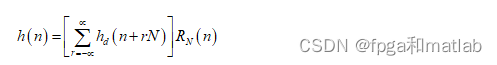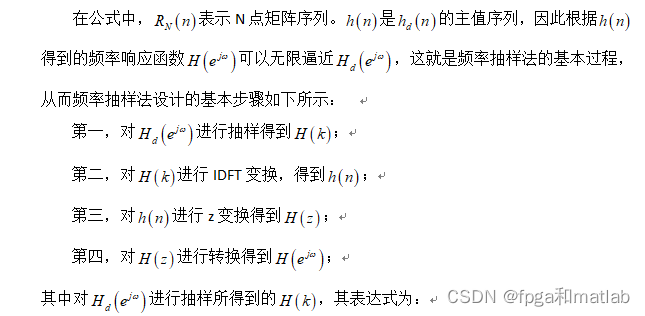# 二、案例背景

滤波器的含义，就是指对输入信号有着消除噪声和平滑功能的系统。根据处理信号种类的不同，将处理连续信号的滤波器称为模拟滤波器，将处理离散信号的滤波器称为数字滤波器。随着通信多媒体技术的飞速发展，数字滤波器成为数字信号处理领域中一个越来越重要的组成部分，其广泛应用在信息通信、语音传输、自适应控制等众多领域。从结构上分，数字滤波器可以分为FIR数字滤波器和IIR数字滤波器两种基本类型。其中，FIR滤波器的设计方法有许多种，如窗函数设计法、频率取样法和等波纹最佳逼近法，本文主要针对FIR滤波器的实现原理和设计方法进行了研究。

数字滤波器本质上讲是一种通过有限精度算法来运算的线性时不变离散系统，其具有特定的传输选择性，数字滤波器的输入和输出信号均为离散数字信号。数字滤波器的工作原理为，通过一个离散数字系统，对输入的数字信号进行运算，处理，变换等各种数字信号操作，从而实现改变数字输入序列的频谱特性或者时域波形，将有用的信号频谱分量进行保留，消除无用的信号频谱分量。数字滤波器，在功能上可以分为低通数字滤波器、高通数字滤波器、带通数字滤波器以及带阻数字滤波器四种类型。相对于模拟滤波器，数字滤波器具有高精度，高稳定性，高灵活性等优势。

# 三、MATLAB核心代码

clc;
clear;
close all;

N = 63;
NN= 31;
H =[ones(1,(N-NN)/2),zeros(1,NN),ones(1,(N-NN)/2)];

k = 0:(N-1)/2;k1=(N+1)/2:(N-1);
A =[exp(-j*pi*k*(N-1)/N),exp(j*pi*(N-k1)*(N-1)/N)];
HK= H.*A;
hn= ifft(HK);

freqz(hn,1,512);
figure;
stem(real(hn),'.');

xlabel('n');
ylabel('h(n)'); 
clc;
clear;
close all;

N = 63;
NN= 51;
H =[ones(1,(N-NN)/2),zeros(1,NN),ones(1,(N-NN)/2)];
k = 0:(N-1)/2;k1=(N+1)/2:(N-1);
A =[exp(-j*pi*k*(N-1)/N),exp(j*pi*(N-k1)*(N-1)/N)];
HK= H.*A;
hn= ifft(HK);

freqz(hn,1,512);
figure;
stem(real(hn),'.');
line([0 35],[0 0]);
xlabel('n');
ylabel('h(n)');

Ts=1/1000  ;%设置采样频率

t=0:Ts:3*pi;
y=sin(2*pi*t)+cos(400*pi*t);
figure;
plotspec(y,Ts);

y_Win=filter(hn,1,y);
figure;
plotspec(y_Win,Ts);



# 四、仿真结论分析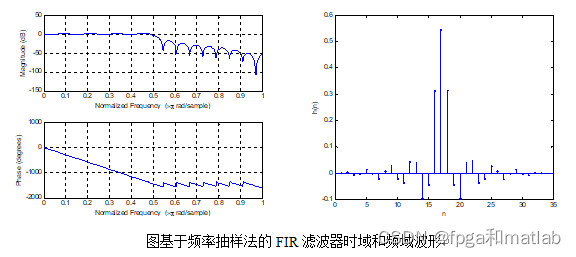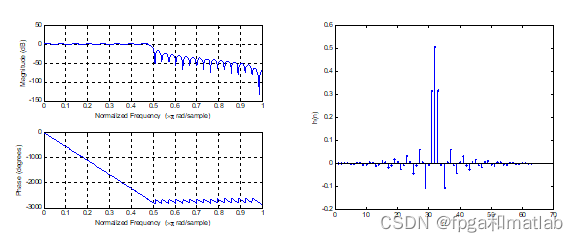从图仿真结果可知，频率抽样法所设计的FIR滤波器，其滤波性能是随着滤波器阶数的增加而增加的，因此，当阶数较大的时候，其硬件实现难度较大。

A25-25

展开全文matlab FIR滤波器
• 如模拟滤波器对连续时间信号的这种运算，在数学上是用线性常系数微分方程确定的。用拉普拉斯变换可在S域内进行这些运算的分析。用傅立叶变换则在f域内分析。同样的，可用常系数线性方程来定义离散时间信号的线性变.....

1 背景

在线性系统中，信号滤波过程一般定义为，当输入波形通过一个系统时，对它作一个线性运算，在时间域上这种变换如像内插，外插微分和积分，在频率域上这种变换则如低通滤波或平滑，带通滤波，谱设计和谱分析。如模拟滤波器对连续时间信号的这种运算，在数学上是用线性常系数微分方程确定的。用拉普拉斯变换可在S域内进行这些运算的分析。用傅立叶变换则在f域内分析。同样的，可用常系数线性方程来定义离散时间信号的线性变换，并且用二变换来分析和合成离散时间滤波器。

## 1.1 数字滤波器的研究背景和意义

当今，数字信号处理技术正飞速发展，它不但自成一门学科，更是以不同形式影响和渗透到其他学科；它与国民经济息息相关，与国防建设紧密相连；它影响或改变着我们的生产、生活方式，因此受到人们普遍的关注。

数字化、智能化和网络化是当代信息技术发展的大趋势，而数字化是智能化和网络化的基础，实际生活中遇到的信号多种多样，例如广播信号、电视信号、雷达信号、通信信号、导航信号等等。上述这些信号大部分是模拟信号，也有小部分是数字信号。模拟信号是自变量的连续函数，自变量可以是一维的，也可以是二维或多维的。大多数情况下一维模拟信号的自变量是时间，经过时间上的离散化（采样）和幅度上的离散化（量化），这类模拟信号便成为一维数字信号。因此，数字信号实际上是数字序列表示的信号，语音信号经采样和量化后，得到的数字信号是一个一维离散时间序列；而图像信号的经采样和量化后，得到的是数字信号是一个二维离散空间序列。

数字滤波技术是数字信号分析、处理技术的重要分支。无论是信号的获取、传输，还是信号的处理和交换都离不开滤波技术，它对信号安全可靠和有效灵活地传输时至关重要的。在所有的电子系统中，使用最多技术最复杂的要算数字滤波器了。数字滤波器的优劣直接决定产品的优劣。

## 1.2 数字滤波器的发展及其现状

数字滤波是从20世纪60年代发展起来的，当时主要采用计算机模拟的方法研究数字滤波问题。到了70年代，开始将微处理器应用于数字滤波。但是由于微处理器速度不高，在很多场合都难以实现实时处理。随着VLSI技术的发展，使用硬件来实现数字滤波器已成为可能。80年代，通用数字信号处理器的成熟和大量涌现，使得音频范围内数字滤波技术迅速得到广泛的应用。近些年来，除了不断提高通用信号处理器的速度和功能外，还出现了许多工作频率达100MHZ以上的专用芯片和积木式部件，从而使数字滤波技术进入视频实时处理阶段。

数字滤波在通信、图像编码、语言编码、雷达等许多领域中有着十分广泛的应用。目前，数字信号滤波器的设计图像处理、数据压缩等方面的应用取得了令人瞩目的进展和成就。近年来迅速发展起来的小波理论，由于其局部分析性能的优异在图像处理中的应用研究，尤其是在图像压缩、图像去噪等方面的应用研究。

## 3 FIR数字滤波器的设计与实现

FIR数字滤波器以其良好的线性特性被广泛应用于现代电子通信系统中，是数字信号处理的重要内容之一。Matlab软件含有多种现成的函数和工具箱,在滤波器设计时只需要调用这些窗函数修改关键数据即可实现不同要求的滤波器设计 。本文主要介绍的是采用窗函数法设计实现FIR带通滤波器。

## 3.1几种常用的窗函数

由上面的分析可知，窗函数法的设计关键是窗函数的选择。实际应用中比较常用的窗函数有：矩形窗函数、三角形((Bartlett)窗函数、汉宁(Hanning)窗函数、哈明(Hamming)窗函数、布莱克曼(Blackman)窗函数和凯塞(Kaiser)窗函数。下面首先介绍这些窗函数的特点。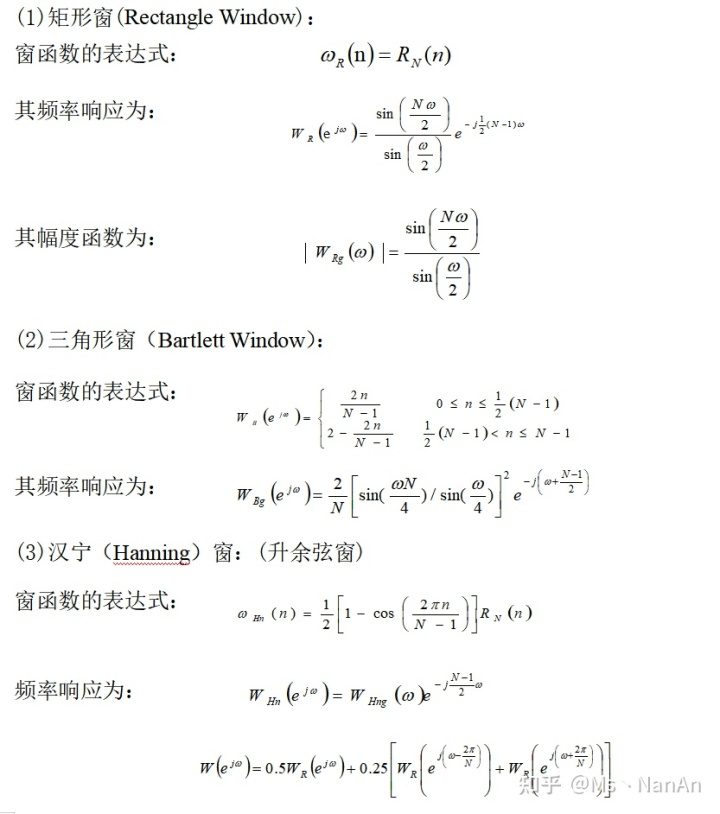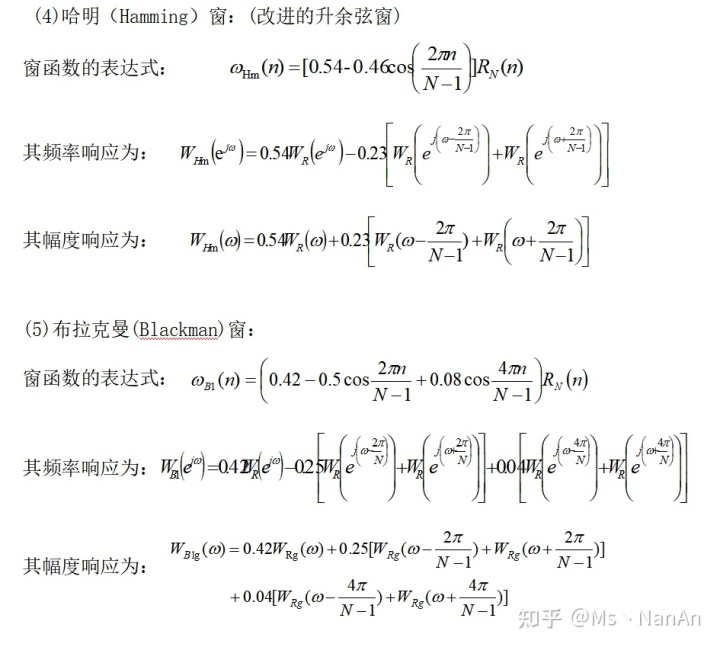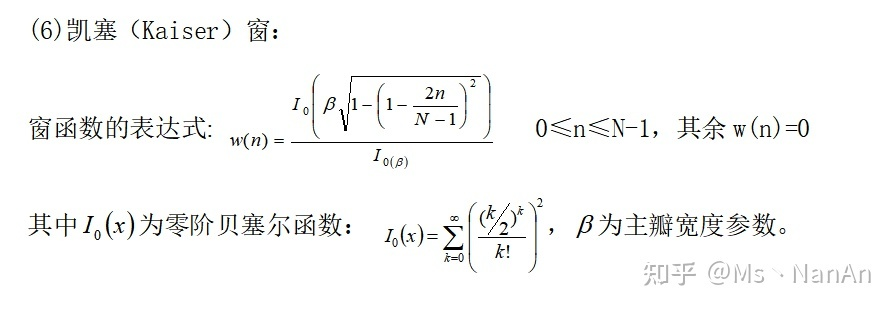这几种窗函数的参数比较如表1所示。

表1　六种窗函数的基本参数

 窗函数 旁瓣峰值幅度/dB 过渡带宽 阻带最小衰减/dB 矩形窗 -13 4π/N -12 三角形窗 -25 8π/N -25 汉宁窗 -31 8π/N -44 哈明窗 -41 8π/N -53 布莱克曼窗 -57 12π/N -74 凯塞窗 -57 10π/N -80

窗函数的选择原则是：

（1）具有较低的旁瓣幅度，尤其是第一旁瓣幅度；

（2）旁瓣幅度下降速度要大，以利增加阻带衰减；

（3）主瓣的宽度要窄，以获得较陡的过渡带。

通常上述三点很难同时满足。当选用主瓣宽度较窄时，虽然得到较陡的过渡带，但通带和阻带的波动明显增加：当选用最小的旁瓣幅度时，虽能得到匀滑的幅度响应和较小的阻带波动，但过渡带加宽。因此，实际选用的窗函数往往是它们的折衷。在保证主瓣宽度达到一定要求的条件下，适当牺牲主瓣宽度来换取旁瓣波动的减少。

## 3.2 利用窗函数设计FIR带通滤波器的设计步骤

本文以带通滤波器的设计为例，介绍窗函数法的实现。用窗函数法设计FIR数字滤波器的步骤如下：

1 根据对阻带衰减及过渡带的指标要求，选择窗函数的类型，并估计窗口长度N。先按照阻带衰减选择窗函数类型。原则是保证阻带衰减满足要求的情况下，尽量选择主瓣窄的窗函数。然后根据过渡带宽度估计窗口长度N。待求滤波器的过渡带宽度近似等于窗函数主瓣宽度，切近似与窗口长度N成反比，N≈，A取决于窗口类型，例如，矩形窗A=4，哈明窗的A=8等。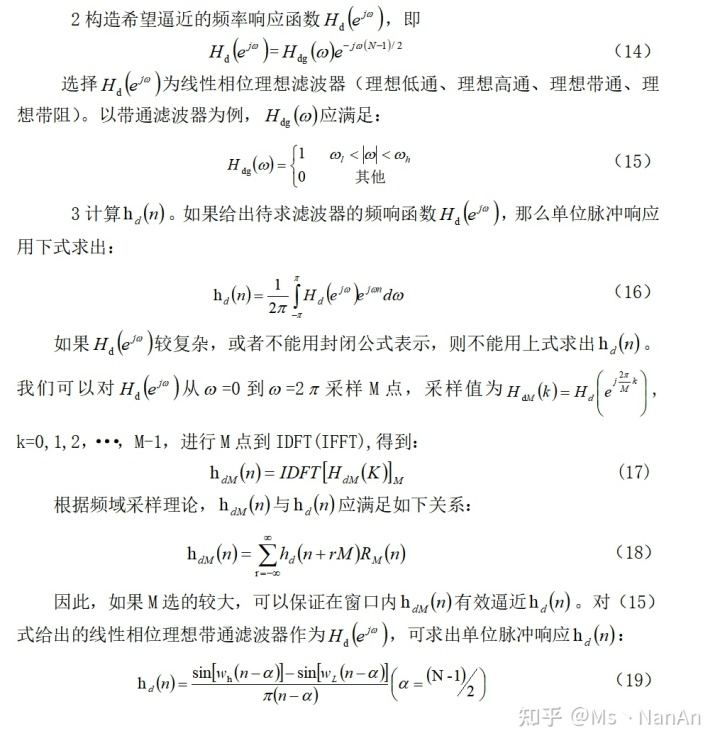## 3.3 基于MATLAB的FIR数字带通滤波器的仿真实现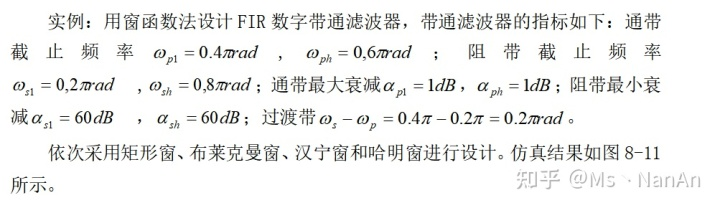实现程序如下：
clear all;close all;clc;
wpl=0.4*pi;wph=0.6*pi;wsl=0.2*pi;wsh=0.8*pi;
wd1=(wpl+wsl)/2;wd2=(wph+wsh)/2;
Bt=min(abs(wsl-wpl),abs(wsh-wph));
N=ceil(11*pi/Bt);
wn=[wd1/pi,wd2/pi];
h=fir1(N-1,wn,'bandpass', boxcar (N)); *// h=fir1(N-1,wn,'bandpass',blackman(N));//
*// h=fir1(N-1,wn,'bandpass',hanning(N));//
*// h=fir1(N-1,wn,'bandpass',hamming(N));//
wd=(wd2-wd1)/2;w0=0.5*pi;
M=(N-1)/2;nn=-M:M;n=nn+eps;
hd=2*sin(wd*n).*cos(w0*n)./(pi*n);           %理想冲激响应
w= boxcar (N)';                   *//w= blackman (N)';//
*//W= hanning(N)';//
*//w= hamming (N)';//
H=20*log10(abs(fft(h,1024)));   % 实际滤波器的分贝幅度特性
HH=[H(513:1024) H(1:512)];
subplot(221),stem(nn,hd,'k');
xlabel('n');title('(a)理想冲激响应');axis([-30 30 -0.4 0.5]);
subplot(222),stem(nn,w,'k');axis([-30 30 -0.1 1.2]);
title('(b)矩形窗');xlabel('n');           *// title('(b)布莱克曼窗');xlabel('n');//
*// title('(b)汉宁窗');xlabel('n');//
*// title('(b)哈明窗');xlabel('n');//
subplot(223),stem(nn,h,'k');
axis([-30 30 -0.4 0.5]);xlabel('n');title('(c)实际冲激响应');
w=(-512:511)/511;
subplot(224),plot(w,HH,'k');
axis([-1.2 1.2 -140 20]);xlabel('\omega/\pi');title('(d)滤波器分贝幅度特性');
set(gcf,'color','w');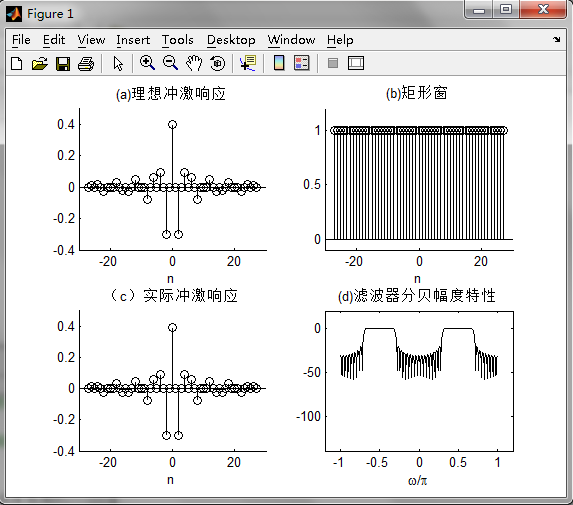图8为选用矩形窗窗设计带通滤波器的仿真结果图。图8（a）表示所要设计的滤波器的冲激响应图，图8（b）为所选用的窗函数——矩形窗。图8（c）为加窗后的实际冲击响应。图8（d）为设计得到的带通滤波器的幅度损耗函数图。由图可以看出，所设计的滤波器具有良好的带通滤波特性，满足设计任务要求。

矩形窗属于时间变量的零次幂窗。矩形窗使用最多，习惯上不加窗就是使信号通过了矩形窗。这种窗的优点是主瓣比较集中，缺点是旁瓣较高，并有负旁瓣，导致变换中带进了高频干扰和泄漏，甚至出现负谱现象。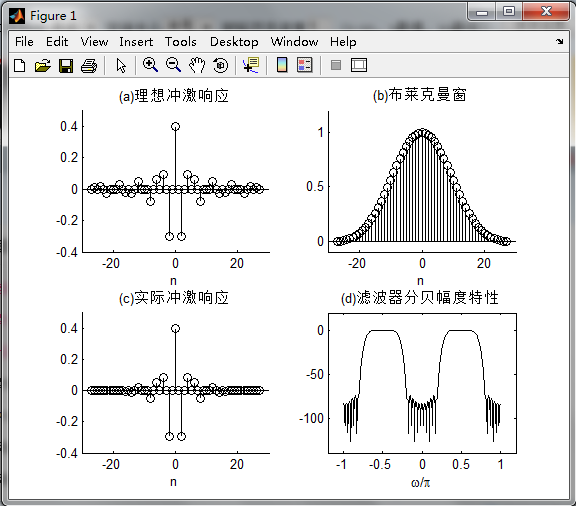图9为选用布莱克曼窗设计带通滤波器的仿真结果图。图9（a）表示所要设计的滤波器的冲激响应图，图9（b）为所选用的窗函数——布莱克曼窗。图9（c）为加窗后的实际冲击响应。图9（d）为设计得到的带通滤波器的幅度损耗函数图。由图可以看出，所设计的滤波器具有良好的带通滤波特性，满足设计任务要求。

布莱克曼窗增加一个二次谐波余弦分量，可进一步降低旁瓣，但主瓣宽度进一步增加，增加N可减少过渡带。布莱克曼窗设计的阻带最小衰减最大，达-74dB，但过渡带最宽，约为矩形窗的三倍。

图10为选用汉宁窗设计带通滤波器的仿真结果图。图10（a）表示所要设计的滤波器的冲激响应图，图10（b）为所选用的窗函数——汉宁窗。图10（c）为加窗后的实际冲击响应。图10（d）为设计得到的带通滤波器的幅度损耗函数图。由图可以看出，所设计的滤波器具有良好的带通滤波特性，满足设计任务要求。

汉宁窗主瓣加宽并降低，旁瓣则显著减小，从减小泄漏观点出发，汉宁窗优于矩形窗．但汉宁窗主瓣加宽，相当于分析带宽加宽，频率分辨力下降。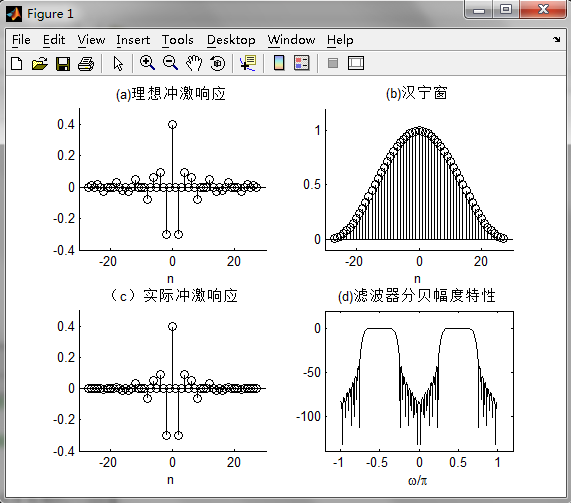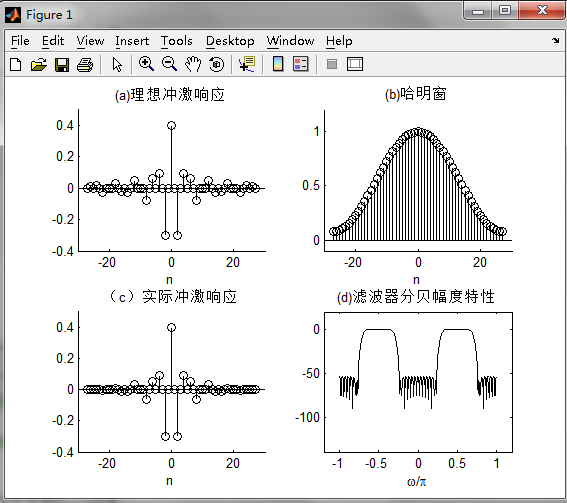图11为选用哈明窗设计带通滤波器的仿真结果图。图11（a）表示所要设计的滤波器的冲激响应图，图11（b）为所选用的窗函数——哈明窗。图9（c）为加窗后的实际冲击响应。图11（d）为设计得到的带通滤波器的幅度损耗函数图。由图可以看出，所设计的滤波器具有良好的带通滤波特性，满足设计任务要求。

哈明窗是对汉宁窗的改进，只是加权系数不同。哈明窗加权的系数能使旁瓣达到更小。在主瓣宽度（对应第一零点的宽度）相同的情况下，旁瓣进一步减小，可使99.96%的能量集中在主瓣内。

## 结论

本文首先介绍了数字滤波器的研究背景及其发展现状，然后介绍了FIR数字滤波器的设计原理。在理解设计方法的基础上，最后基于MATLAB软件利用窗函数法实现了FIR数字带通滤波器的设计。仿真结果表明，所设计的滤波器具有良好的滤波器特性，所设计的指标符合设计任务要求。

FIR数字滤波器的设计要取得好的逼近效果，除阶数N外，选择适合的窗函数也是重要方面的。通过对窗函数合理的选择，使得滤波器的通带衰减较小，阻带衰减较大；有较陡的过渡带，有利于对信号频带的选择。

在FIR滤波器中突出的性能中，也存在着一些不足：

1. 窗函数设计法是从时域出发的一种设计法，但一般技术指标是在频率给出的，其边界频率不容易控制，为达到较好的效果，需要多次调试。

2. 由于FIR滤波器的传输函数的极点固定在原点，所以只能采用较高的阶数来达到高的选择性。因此，它的经济性就相对差一些。

MATLAB由于本身带有多种工具箱和窗函数对于滤波器的设计有着多种设计方式，只需修改关键程序即可实现多种滤波器的设计。在图像处理、通讯、控制系统设计、测试和测量、财务建模和分析以及计算生物学等众多应用领域有着不可替代应用前进。

展开全文python 机器学习 java 人工智能
• 先进入filterDesigner，老版本的是fdatool。滤波器参数设置如下图所示。Specify Order设置为15...Matlab代码如下： 运行结果如下： 滤波器中1.768kHz时，为-3dB点，而在上图时域中可以看出，输出信号的幅值下降到0.70

先进入filterDesigner，老版本的是fdatool。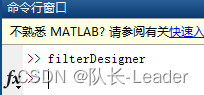滤波器参数设置如下图所示。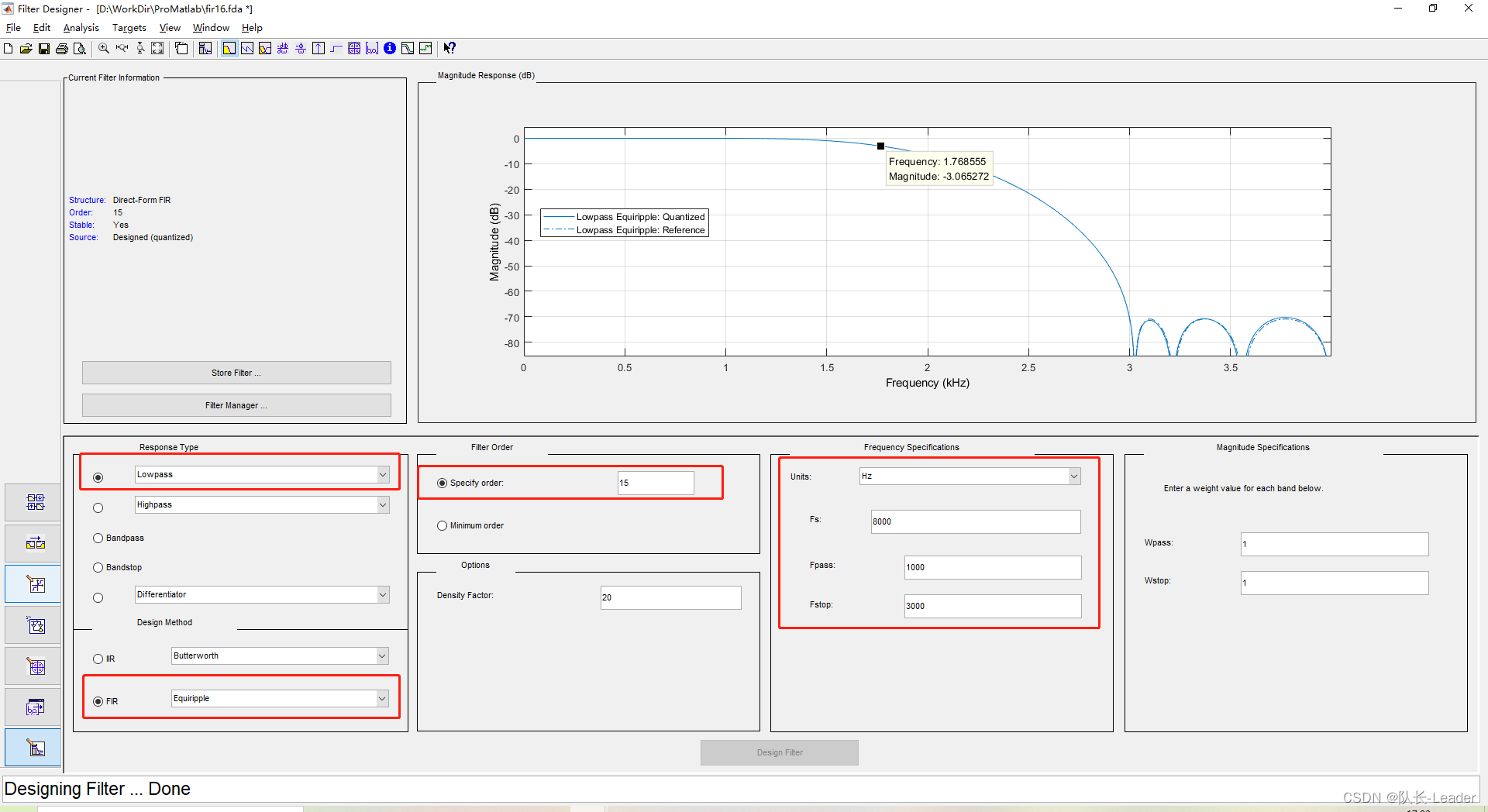Specify Order设置为15，实际阶数为16阶。

滤波器参数为：等纹波滤波器，低通，采样率8kHz，通带频率为1kHz，阻带频率为3kHz。

点击Design Filter，即生成滤波器的幅频特性曲线。

量化参数设置如下图所示。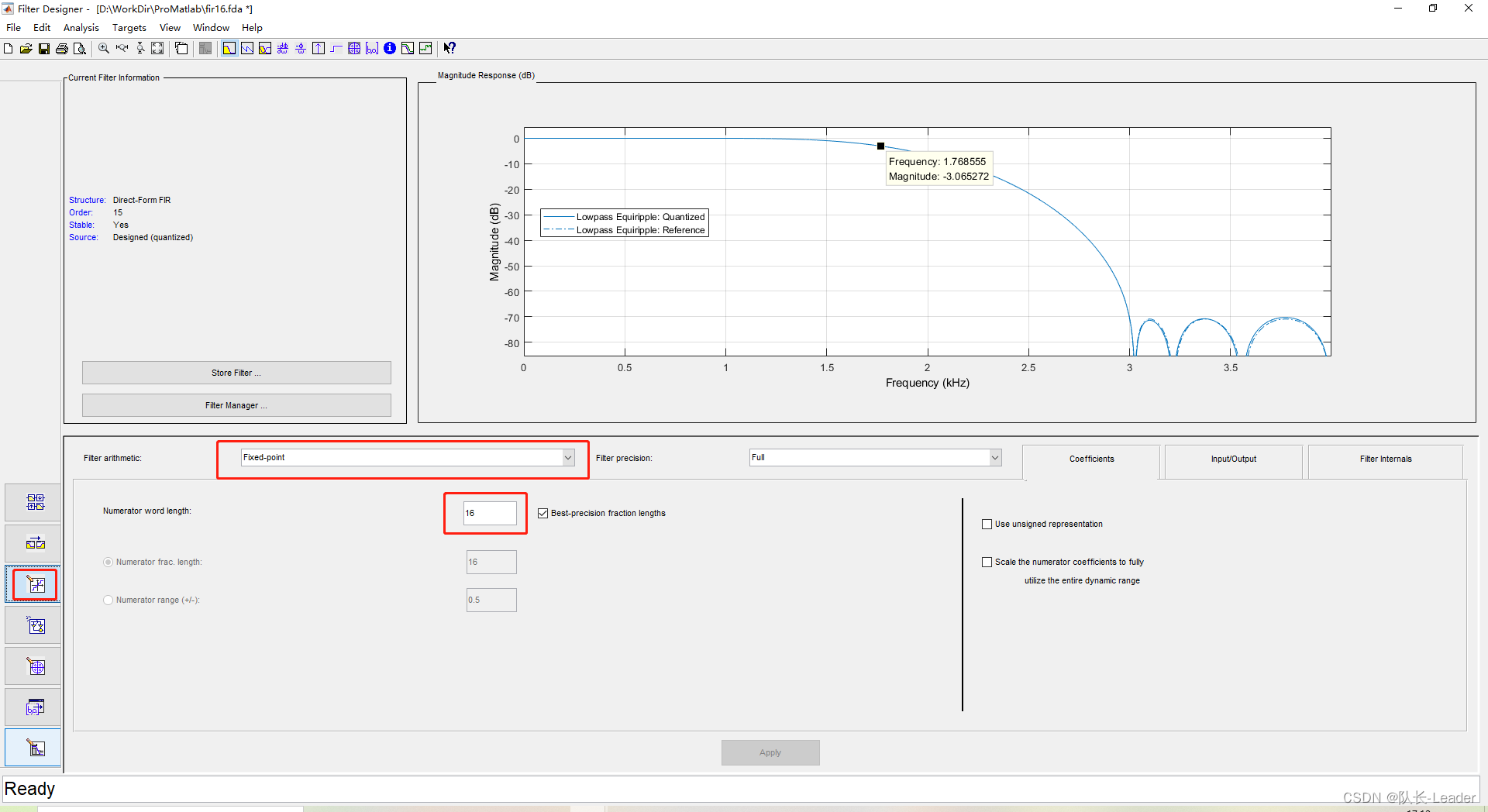生成c头文件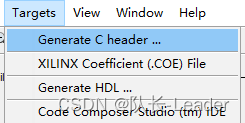从该头文件件中，复制出滤波器的系数出来。

Matlab代码如下：

close all; clear all; clc;
coe =[-122 -223 743 1190 -2645 -4306 9187 28935 28935 9187 -4306 -2645 1190 743 -223 -122]; %滤波器系数

len = length(coe); % 滤波器阶数
Fs=8000; % 采样率
T=0.1; % 时间
dt=1.0/Fs; % 时间刻度
N=T/dt; % 点数
t=linspace(0,T,N); % 时间向量
x = sin(2*pi*1768*t); % 输入信号,1768Hz
outputY = conv(x,coe)/(2^len); % 输出信号

subplot(211)
plot(t,x);
subplot(212)
plot(outputY)

运行结果如下：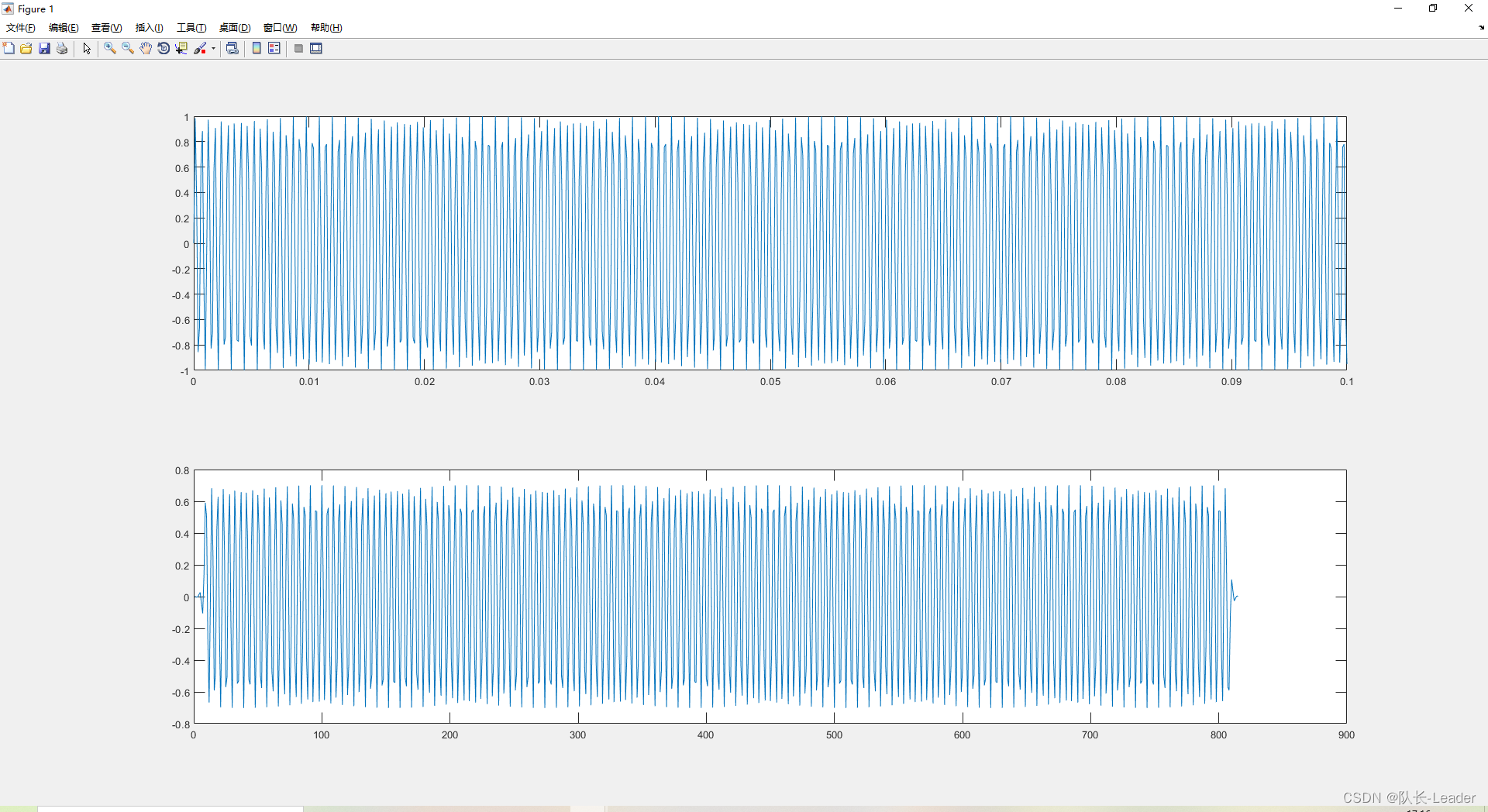滤波器中1.768kHz时，为-3dB点，而在上图时域中可以看出，输出信号的幅值下降到0.707倍。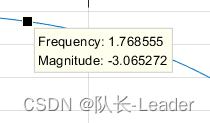展开全文matlab
• MatlabFFT频谱分析及FIR数字滤波器-滤波器.rar 最近在做关于滤波器方面的课题，搜集了一些资料，为了不浪费大家的M币，我将6篇PDF文档压缩一起了，里面伴有程序和详细说明，希望会对大家有帮助！ 资料有：1.FFT....matlab
• FIR滤波器设计文献集-基于MATLABFIR数字滤波器的设计.pdf 本帖最后由 zyzhang 于 2012-4-24 18:52 编辑 载自各大数据库希望能帮到大家 基于Matlab的FIR带通滤波器的设计与仿真.pdf 基于Matlab...matlab
• FIR滤波器由于没有反馈，比较不稳定，消耗资源比较多，但是却可以产生线性相位。 线性相位有什么用， 然后我简单说一下FIR滤波器原理（图说搬来的），原理很简单，我们设计一个低通滤波器，就是用一个窗函数去截...matlab
• 本书以Altera公司的FPGA器件为开发平台，采用MATLAB及Verilog HDL语言为开发工具，详细阐述数字滤波器的FPGA实现原理、结构、方法以及仿真测试过程，并通过大量工程实例分析FPGA实现过程中的具体技术细节。...
•matlab
• FIR数字滤波器程序代码转载-85375517Digital_signal_Process.rar 数字信号处理 各种滤波器的设计，中南大学电子信息工程 大三的课程设计全部源代码和实验报告，欢迎下载！-various digital signal processing ...matlab
• matlab设计FIR高通滤波器，内涵实例，代码
• MATLAB实现巴特沃斯数字滤波器前因：因为要准备保研面试，今年暑假就重新把烂尾的项目捡起来了。为了提取采集到的脑电信号中有用的部分，想用数字带通滤波器实现，浏览了很多帖子。要不是只有代码，没有注释；要不...
• 根据处理信号种类的不同，将处理连续信号的滤波器称为模拟滤波器，将处理离散信号的滤波器称为数字滤波器数字滤波器的工作原理为，通过一个离散数字系统，对输入的数字信号进行运算，处理，变换等各种数字信号操作...matlab FIR滤波器
• 基于MATLABFIR数字滤波器的设计.pdf 基于MATLABFIR数字滤波器的设计 基于MATLAB的频率采样法设计FIR滤波器.pdf 基于MATLAB的频率采样法设计FIR滤波器 基于频率...matlab
• 数字信号处理实验，包含代码跟实验截图，注释清晰明了，实验结果正确matlab
• Matlab数字滤波器的设计（IIR与FIR)（内含Matlab代码FIR滤波器的优缺点 优点：相位线性度好，处理速度快，没有反馈回路稳定性强于IIR。 缺点：FIR幅频特性精度较之于IIR低 IIR滤波器 优点：相同阶数下IIR滤波效果...matlab
• 摘要：提出FIR敷字滤波器的设计方案，并基于Matlab实现滤波仿真。通过使用Matlab信号处理工具箱提供的函数，选择适当的窗函数编写程序，其中窗函数按照实际信号的处理需求，参数折中选择。实验获得了比较理想的...
• 基于MATLABFIR数字滤波器的设计.pdf 基于MATLABFIR数字滤波器的设计 基于MATLAB的频率采样法设计FIR滤波器.pdf 基于MATLAB的频率采样法设计FIR滤波器 基于频率...matlab
• 基于CCS环境和MATLAB仿真的FIR数字滤波器实现.pdf
• 本压缩包内包含了FIR数字滤波器设计的基本所有代码，窗函数（Hamming窗、Blackman窗、Kaiser窗等），包含了频率采样法和窗函数法设计FIR滤波器的代码
• matlab代码仿真设计的导通低通带通带阻四种fir数字滤波器，亲测可用，并且做了非常详细的代码说明，
• 文件是用matlab设计的fir数字滤波器，包括带通、带阻、低通、高通、低通加带阻五种滤波器，代码都是自己编写并且验证通过，代码中有非常详尽的说明，每行代码基本都有注释，对于学习用matlab设计fir数字滤波器有很大...
• 八、实验心得1、对于MATLAB语句有了更加深刻的理解，也注意到了一些运算符号的使用，例如数组的相乘需用(.*)来表示，而一般数字相乘应用*。...4.设计过程中，学习了许多数字信号处理课程中关于数字滤波器的...
• FIR滤波器matlab仿真/数字滤波器...6、matlab设计的五种fir数字滤波器 7。MMTLAB实现数字PIR的高通和带通等滤波器的源程序 8、matlab实现小波去唾，维纳滤波去项以及自进应月R谁波算程序员帮帮 9、MATLAB话音处理，语音matlab
• 随着信息时代和数字世界的到来，数字信号处理已成为今一门极其重要的学科和技术领域。数字信号处理在通信、语音、图像、自动控制、雷达、军事、航空航天...在数字信号处理应用中，数字滤波器十分重要并已获得广泛应用。...